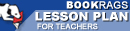Lesson Plans

# Journey Through Genius: The Great Theorems of Mathematics Quiz | Four Week Quiz B

This set of Lesson Plans consists of approximately 142 pages of tests, essay questions, lessons, and other teaching materials.
 View a FREE sampleName: _________________________ Period: ___________________

This quiz consists of 5 multiple choice and 5 short answer questions through A Sampler of Euler's Number Theory.

## Multiple Choice Questions

1. Dunham showed that Heron's proof could also be used as which of the following?
(a) A proof of Euclid's number theory
(b) A proof of Archimedes' number theory.
(c) A proof of the Pythagorean Theorem.
(d) A proof of Hippocrates' squared areas.

2. Who wrote a treatise that supposed that cubic equations may be impossible to solve?
(a) Gerolamo Cardano.
(b) Scipione del Ferro.
(c) Luca Pacioli.
(d) Niccolo Fontana.

3. What was the same about the series proposed by Leibniz and the series proposed by Bernoulli?
(a) Both series were divergent.
(b) Both series were composed of successively smaller terms.
(c) Both series were composed of successively larger terms.
(d) Both series were convergent.

4. Besides being a mathematician, what else other work was Archimedes famous for?
(a) Doctor and writer,
(b) Inventor and scientist.
(c) Politician.
(d) Artist and musician.

5. What did Gauss do with his best work?
(a) He gave it to his son to publish.
(b) He published it.
(c) He did not publish it.
(d) He gave it to his students.

## Short Answer Questions

1. What did Dunham consider as Archimedes's "masterpiece"?

2. What was true about Heron's theorem as described by Dunham?

3. What great theorem is presented by Dunham in this chapter?

4. What did Gauss construct?

5. Which of the following was a major part of Gauss' work in mathematics?

(see the answer key)

 This section contains 398 words (approx. 2 pages at 300 words per page) View a FREE sampleCopyrightsJourney Through Genius: The Great Theorems of Mathematics from BookRags. (c)2019 BookRags, Inc. All rights reserved.
Follow Us on Facebook